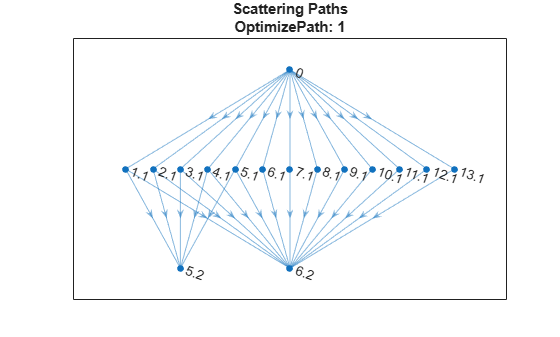# paths

Scattering network paths

## Syntax

``spaths = paths(sf)``
``[spaths,npaths] = paths(sf)``

## Description

````spaths = paths(sf)` returns the scattering paths for the scattering network, `sf`. `spaths` is a NO-by-1 cell array of MATLAB® tables, where NO is the number of orders in the network.```

example

````[spaths,npaths] = paths(sf)` returns the number of wavelet scattering paths by order. `npaths` is a NO-by-1 vector, where NO is the number of orders in the scattering network. The ith element of `npaths` contains the number of scattering paths in the (i-1)th order.```

## Examples

collapse all

Create two wavelet scattering networks, both for a signal of length 500. In the second network, set the `OptimizePath` value to `true`.

```sf = waveletScattering('SignalLength',500); sfOpt = waveletScattering('SignalLength',500,'OptimizePath',true);```

Obtain the path information of both networks. Determine the total number of scattering paths in both networks.

```[spaths,npaths] = paths(sf); [spathsOpt,npathsOpt] = paths(sfOpt); str = sprintf('Paths in default network: %d\nPaths in path-optimized network: %d\n',... sum(npaths),sum(npathsOpt)); fprintf(str)```
```Paths in default network: 65 Paths in path-optimized network: 52 ```

Both networks have two filter banks. Visualize the scattering paths that include the wavelets in the second filter bank. Create a directed graph. For every wavelet filter that is on at least one path, label the corresponding node as waveletNumber.filterbank. For each path, connect the corresponding nodes. Use the helper function `helperPlotScatteringGraph` to construct the graphs. Plot the graphs of both networks.

```scatGraph = helperPlotScatteringGraph(spaths); plot(scatGraph) title({'Scattering Paths',['OptimizePath: ',num2str(sf.OptimizePath)]})``````figure scatGraphOpt = helperPlotScatteringGraph(spathsOpt); plot(scatGraphOpt) title({'Scattering Paths',['OptimizePath: ',num2str(sfOpt.OptimizePath)]})```Supporting Functions

plotScatteringGraph

```function dirGraph = helperPlotScatteringGraph(networkPaths) % This function is intended for use only in this example. It may change or % be removed in a future release. path = networkPaths{3}.path; % set to 0 if want to show the multiple paths between 0 and each % first level node mkunique = 1; if mkunique == 1 f1 = path(:,1:2); c = unique(f1,'rows'); else c = path(:,1:2); end p1 = string(c(:,1)); p2 = string(c(:,2)+.1); p3 = string(path(:,2)+.1); p4 = string(path(:,3)+.2); dirGraph = digraph([p1;p3],[p2;p4]); end```

## Input Arguments

collapse all

Wavelet time scattering network, specified as a `waveletScattering` object.

## Output Arguments

collapse all

Scattering paths, returned as a NO-by-1 cell array of MATLAB tables, where NO is the number of orders of the scattering network.

Each MATLAB table in `spaths` contains three variables:

• `path` — Scattering network paths. In the kth element of `spaths`, `path` is a N-by-k matrix where each row contains a path from the input data through the (k-1)th wavelet filter bank. For example, when k equals 1, N is equal to 1 and the only path is 0 denoting the input data. When k equals 2, N is equal to the number of wavelet filters in the first filter bank and `path` is a N-by-2 matrix describing the path from the input data, 0, through the wavelet filters in the first filter bank. The second column of `path` contains the wavelet filters in the first filter bank ordered by decreasing center frequency.

• `log2ds` — The incremental base-2 log downsampling factor for the scalogram coefficients corresponding to the cumulative path in the same row.

• `log2res` — The base-2 log resolution of the scalogram coefficients corresponding to the cumulative path in the same row.

Number of wavelet scattering paths in the network by order, returned as a vector. `npaths` is a NO-by-1 vector where NO is the number of orders in the network. The ith element of `npaths` contains the number of scattering paths in the (i-1)th order. The sum of the elements of `npaths` is the total number of scattering paths.

## Version History

Introduced in R2021a